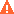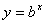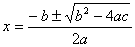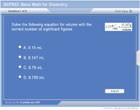HOME COURSES PREVIEW REVIEW ABOUT
 TOLL-FREE INFO & ORDERING: M-F: 9am-5pm (PST): (877) RAPID-10
 QUICK TOUR: Quick Movie - How to Learn in 24 Hours
 QUICK SEARCH: Keywords:
 Rapid Learning Member Area:Note: If you are a legacy user of chemistry24 members, please request a new login access to the premium server with your full name and old login email via vip@rapidlearningcenter.com
 RAPID COURSE CATALOG: Chemistry in 24 Hours
CHEMISTRY TIPS WEEKLY:

Are you taking a chemistry course or preparing for a chemistry exam? If you are learning chemistry now, the Chemistry Tips Weekly is for you--it is a one minute learning each week to the chemistry mastery, free for all students. The chemistry tips will include the follwing topics

• Chemistry survival basics
• Top ten confusions in chemistry
• How to ace chemistry exams
• Problem-solving tips in chemisrty
• Plus much more...
Enter your name and email address below and click "Subscribe" to have free Chemistry tips delivered to your inbox weekly.

 OTHER RELATED SITES: ChemistrySurival.com BiologySurvival.com Biology24.com Physics24.com Mathematics24.com RapidLearningCenter.com
 Note: For course links to launch, disable popup blockers or hold the ctrl key while clicking the link.Bonus - Free Coaching for 3 Months :  Need help on your homework or exam questions? Get stuck on chemistry problems? Let Dr. Wayne and his team of faculty help you personally. Order one of our 24-hour rapid chemistry courses today and you will receive 3-month chemistry coaching (\$297 value - free). Limited to first 250 students only. Grab yours today!

Basic Math for Chemistry

 Topic Review on "Title": Math skills are needed throughout a chemistry course. Algebra Algebra is used to solve equations by un-doing whatever is being done to an unknown variable. For example, if an equation has “x+2” then you would subtract “2” to solve for “x”.  Everything that is done to one side must be done to the other side of the equation as well. Calculations with significant figures You cannot become more precise after completing calculations than the original data was.  Therefore, it is important to write the answer with the correct number of significant figures.  When adding and subtracting with significant figures, you write the answer with the least number of decimal places that are in the problem.  When multiplying and dividing, write the answer with the least number of significant figures as is in the problems. Scientific Notation Scientific notation is a way of writing large or small numbers as a multiple of 10.  The decimal place is always placed behind the first non-zero number and the number of times the decimal point was moved to get there is used as the exponent of 10.  Positive exponents represent large numbers (>1) and negative exponents represent small numbers (<1). Exponents Several rules are used for exponents: Anything to the 1st power = the original number Anything to the power of 0 = 1 When multiplying numbers with exponents, if the #’s are the same, you can add the exponents and put the total with the original number When dividing numbers with exponents, if the #’s are the same, you can subtract the exponents and put the difference with the original number When taking a power to a power, multiply the powers A negative power indicates that it’s on the opposite side of the fraction There are similar rules when working with scientific notation numbers: Addition with same powers of 10:  Add the numbers and keep the power of 10 the same. Subtraction with the same powers of 10:  Subtract the numbers and keep the power of 10 the same. Multiplication: Multiply the numbers and add the powers of 10 Division: Divide the numbers and subtract the powers of 10 Power: Take the number to that power and multiply the power of 10 by the power Roots:  Take the root of the number and divide the power of 10 by the root Logarithms Logarithms are a way of counting in multiples of a base number.  Ifthen.  If no base is specified, it’s assumed to be 10.  A natural log (ln) uses the base of “e” (2.313). Quadratic Formula The quadratic formula is used to solve for a variable when there is the variable to the 2nd power and to the 1st power in the same equation.  IfthenCalculator Tips People often get incorrect answers simply from a mistake in the way they enter numbers into their calculator.  When dividing by more than one number, use the ¸ button each time.  When entering scientific notation, always use the EE (or EXP) button rather than entering (^10).  Be sure to use parenthesis around addition and subtraction when combining with multiplication and division, and also when taking a value (especially a negative value) to a power.

Rapid Study Kit for "Title":
 Flash Movie Flash Game Flash Card Core Concept Tutorial Problem Solving Drill Review Cheat Sheet"Title" Tutorial Summary : Math skills are crucial throughout chemistry.  This tutorial reviews basic algebra needed in chemistry calculations.  Writing answers with the correct number of significant figures is taught, along with writing and reading numbers in scientific notation.  Performing calculations with exponents, including those in scientific notation, is illustrated.  Logarithms, both base 10 and natural logs, is taught.  The quadratic equation is demonstrated.  Finally, calculator survival tips are given to ensure the answer you type in what you intend.  All mathematical concepts are illustrated with chemistry applications—each one is actually used in chemistry calculations.

 Tutorial Features: Specific Tutorial Features: Examples of the math techniques use chemistry applications Series Features: Concept map showing inter-connections of new concepts in this tutorial and those previously introduced. Definition slides introduce terms as they are needed. Visual representation of concepts Animated examples—worked out step by step A concise summary is given at the conclusion of the tutorial.

 "Title" Topic List: Algebra Solving for a variable in addition/subtraction and multiplication/division Calculating with significant figures Addition/subtraction Multiplication/division Scientific Notation Writing in scientific notation Reading scientific notation Exponents rules Calculations with exponents Calculations with scientific notation Logarithms Logarithms with base 10 Natural logarithms Quadratic Equation Calculator tips

See all 24 lessons in college chemistry, including concept tutorials, problem drills and cheat sheets:
Teach Yourself College Chemistry Visually in 24 Hours

 © 2015 Rapid Learning Center | Privacy Policy | Disclaimer | Affiliates Chemistry Survival, Biology Survival, Physics Survival, and Mathematics Survival Publishing are the divisions of Rapid Learning Inc.